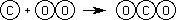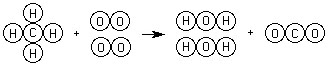Chemical Reactions - General Information

## Chemical Reactions - General Information

Chemical equations are a shorthand method of representing chemical reactions. In a chemical reaction the reactants (what you start with) are converted into products (what you end with). The reactants, which are shown on the left hand side of an equation, and the products, which are shown of the right, are separated by an arrow. The equation below represents the reaction of carbon with oxygen gas to form carbon dioxide.

C + O2CO2

This reaction could also be represented pictorially:Notice that the number of atoms of carbon is the same on both sides of the arrow. There is one carbon atom on the reactant side and one carbon atom on the product side. The same is true for oxygen except that there are two oxygen atoms on each side (remember that the subscript of two in the oxygen molecule means that there are two oxygen atoms bonded together). When the number of atoms on each side of the equation are equal, the equation is said to be balanced. A balanced equation is in agreement with the Law of Conservation of Matter which states that matter is neither created nor destroyed during a chemical reaction.

When methane (CH4) reacts with oxygen it forms carbon dioxide and water.

CH4 + O2H2O + CO2

Here we notice that the reaction is not balanced as the number of hydrogen atoms are different on each side. The same is true for the number of oxygen atoms. In order to balance this equation we must add coefficients in front of oxygen and water. Coefficients are used when we want to represent more than one of a particular atom or molecule.

CH4 + 2 O22 H2O + CO2

With the addition of the coefficients the reaction is balanced. One methane molecule reacts with two oxygen molecules to form one carbon dioxide molecule and two water molecules. Pictorially this could be represented by:Often the physical state of the reactants or products is also included in an equation: (s) is used for a solid, (l) for a liquid and (g) for a gas. If a substance is dissolved in water an (aq), which stands for aqueous, is used. Adding these symbols, the equation becomes:

CH4(g) + 2 O2(g)2 H2O(l) + CO2(g)

A number of other symbols are sometimes used in writing chemical equations.

Continue and read about how to classify reactions.